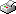Print This Page

Ellinor Fackle Fornius

-- licentiate thesis --

##### Optimal Design of Experiments for the Quadratic Logistic Model

Abstract
Optimal design of experiments for binary data is the topic of this thesis. A particular logistic model containing a quadratic linear predictor and one control variable is considered. The problem that the optimal designs for this model depends on the unknown true parameters is in focus. The aim of the first paper is to examine the small sample performance of the optimal designs and to investigate the loss from using non-optimal designs. D-optimal designs are derived for different parameter sets and compared to a couple of non-optimal designs in a simulation study. The evaluations are made in terms of mean squared error of the maximum likelihood parameter estimator. Another problem with this model is the occurrence of certain data patterns for which no maximum likelihood estimates can be obtained. The designs differed considerably in this respect and this problem also turned out to be parameter dependent. When it comes to the small sample distribution of the maximum likelihood estimator it was demonstrated to be quite different from the asymptotic distribution. The aim of the second paper is to find a solution to the parameter dependence issue. Two sequential approaches are proposed and tested in a simulation study. The purpose is to .nd the conditions that maximize the probability of response. The first approach is an optimal design approach where c-optimal designs are updated sequentially. The second approach is a stochastic approximation approach which is a nonparametric approach, that is no distribu- tional assumptions has to be made. The two approaches are compared in terms of mean squared error. Based on the simulation results the c-optimal design approach was consistently favored.